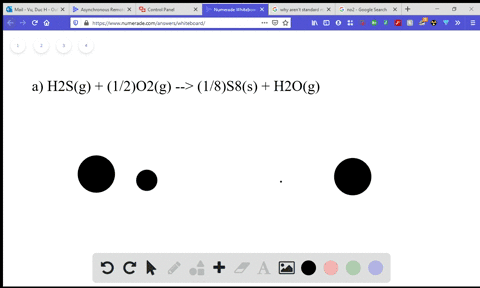Enroll in one of our FREE online STEM summer camps. Space is limited so join now!View Summer Courses### Without using Appendix $\mathrm{B}$ , predict the…

06:04North Carolina State University

Need more help? Fill out this quick form to get professional live tutoring.

Get live tutoring
Problem 16

Without using Appendix $\mathrm{B}$ , predict the sign of $\Delta S^{\circ}$ for
(a) $2 \mathrm{K}(s)+\mathrm{F}_{2}(g) \longrightarrow 2 \mathrm{KF}(s)$
(b) $\mathrm{NH}_{3}(g)+\mathrm{HBr}(g) \longrightarrow \mathrm{NH}_{4} \mathrm{Br}(s)$
(c) $\mathrm{NaClO}_{3}(s) \longrightarrow \mathrm{Na}^{+}(a q)+\mathrm{ClO}_{3}^{-}(a q)$

Check back soon!

## Discussion

You must be signed in to discuss.

## Video Transcript

Dr 20 Problems 16 is asking us to predict the sign of entropy. Four of reaction. So first us were caught. Attribute is the freedom of part of motion or the dispersion of entropy. The greater the entropy, the greater the freedom of particle motion or the grave that dispersion. So in this case, we have an increase. Intra, Pete, when we go from the solid to a liquid to the gas date gas particles have a greater freedom of particle motion, a greater dispersion, entropy, and therefore they have a greater entropy. There's something that's a liquid and liquid also has greater than something that's a solid. So we can use this change and phase to make predictions about a reaction, whether or not we're gonna have ah greater changing s or a smaller change nets. So a change in s is shown by the final entropy, minus the initial entropy. It's the entropy at the end, subtracting that from the intra Pete in the beginning, If that value is negative, then we'd have a negative change. Inter, Pete. If that value is positive, we have a positive change of entropy. Let's consider reaction where we're working with to Mosul X. The actual substance of X is not too important now. But what is important is that it's in the solace t and we're combining that with one mole of why Why happens to be Die atomic in this case and why's in its gas state? We combine these two, we end up with two moles of X y, and this product is also in its solid state. So we begin with three moles of a substance and we end with two moles of a substance. In addition, one of those substances that we begin with this gas and gas has a large inter p a large free of a part of commotion. So in the beginning, our entropy is greater than in the end. So we are doing a small value minus a larger value. So in the end, again, we take our our final entropy, which in this case is small. So we'll take a small value and we're going to subtract Ah, larger value from the entropy. In the beginning, because we had a gas was had a high entropy. So this total entropy here would be larger. A small value minus a large value is going to give us a negative number. And so in this case, are entropy of the system. We could predict it to be negative. Let's consider another reaction where we have one mole of X and this is a gas. We combine this one mole of X with one mole of why and this wise also a guest. So we know because they're gases, that the initial entropy is large. Two moles of gases and they're both large have large entropy is we produce X Y and X Y ends up being a solid. So again you notice that the entropy at the end is lower than the entropy at the beginning, because a solid product solid substance has ah smaller contributing a larger. So we took our solid, which is a small entropy. Subtract that from a gas which has a large entropy, and again we're gonna end up with a negative Delta s. Lastly, let's look at something that began as a solid and we combine This actually didn't combine the solid with anything. That's how it broke down into two substances. Let's make this salad eggs. Why? And it broke down into X, which is a curious which means it's in a liquid state and it broke down until why which is also angriest and for the sake of continuity will include the charges here. Okay, so we had X y broke down into X, and why both of these air quiz? So here we're going from something that's a solid that we know has an initial small freedom of particle motion, a small entropy, and we're ending up something that's a liquid that has more energy. So our final is a liquid which has a larger energy. We're subtracting that from a solid which has a smaller state of energy, and so a large number of modest a small number is going to give us a positive entropy here. So to recap, the SS here are representing small en trapeze versus the L's, which are representing large n trapeze. And when you go from something that has a small entropy to something that has a large entropy, your initial was small. Your final is large, large, minus a small entropy will give you a positive change of entropy. What if you began with a large entropy and then it ended up decreasing to a smaller entry? That change will be negative, giving you a negative entropy of that system